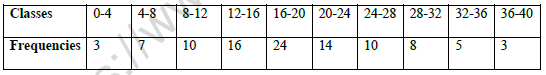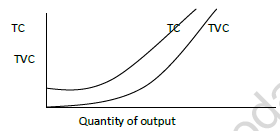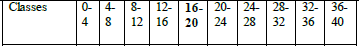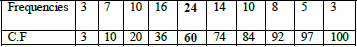# CBSE Class 11 Economics Worksheet Set H Solved

Download printable Economics Class 11 Worksheets in pdf format, CBSE Class 11 Economics Worksheet Set H Solved has been prepared as per the latest syllabus and exam pattern issued by CBSE, NCERT and KVS. Also download free pdf Economics Class 11 Assignments and practice them daily to get better marks in tests and exams for Grade 11. Free chapter wise worksheets with answers have been designed by Standard 11 teachers as per latest examination pattern

## Economics Worksheet for Class 11

Class 11 Economics students should refer to the following printable worksheet in Pdf in Grade 11. This test paper with questions and solutions for Standard 11 Economics will be very useful for tests and exams and help you to score better marks

### Class 11 Economics Worksheet Pdf

SECTION A

1.When Average Product Curve coincides with Marginal Product Curve, Average Product Curve is:

A. Constant and maximum
B. Constant and minimum
C. Increasing at a constant rate
D. Increasing at a diminishing rate

2.A firm increases its output 20 to 40 units. As a result its Total Cost rises from Rs.2000 to Rs.2400. What could be the marginal cost of production at 40th unit?

3.As firm increases the output, the difference between Average Cost and Average Variable cost diminishes due to:

A. As output increases Average cost diminishes.
B. As output increases Average Variable cost diminishes
C. As output increases Average Fixed cost diminishes
D. All the above

4.Using suitable diagram distinguish between Total Cost and Total Variable cost. 3

5.Consider the following production schedule of a firm. Identify and explain the three stages of Law of Variable Proportion using the values derived from the table.SECTION B

6.Give one important property of Arithmetic Mean.

7.‘Captions’ in a table represent:

A. Title of the table
B. Title given to columns in the table
C. Title given to the rows in the table.
D. Title for sources of data given in the table.

8.How a Multiple Bar Diagram is is different from a Histogram.

9.Construct a Multiple Bar Diagram for the following data related to construction of a house in New Delhi.10. Calculate median for the following distribution. Locate the value on a graph.SET - C

VALUE POINTS

1. A. Constant and Maximum

2. Rs. 20

3. C. As out increases Average Fixed Cost diminishes

4 Total cost is the sum of cost of fixed inputs and variable inputs. TVC is cost incurred on variable inputs only TVC is zero at zero unit of output. TC is equal to TFC at zero unit of output5.Stage I: Up to 3rd unit of input, MP increases. TP increases at an increasing rate. Firm is in the stage of increasing returns

Stage II: From 4th unit to 8th unit of input, MP decreases but positive. TP increases at a diminishing rate. At 8th unit of input MP is zero TP reaches maximum and constant. Firm is in the stage of diminishing returns.

Stage III: Beyond 8th unit, MP is negative. TP falls. Firm is in the stage of negative returns.

SECTION B

6 The sum of deviation taken between the values and its mean will be zero

7 B. Title given to columns in the table

8 Multiple bar diagram consist of different sets of rectangles of equal width. Histogram is a set of rectangles of different width according to width of the classes (any one difference)

Multiple bar diagramDiagram

10M = (N/2) th item; 100/2 = 50th item
Median class="16-20"
M = L + (N/2-CF/f) x h
= 16 + (50-36)/24 x 4
= 16 = 14/24 x 4
= 14 + 2.3 =16.3
Ogive and location
On graph 16.5 approximate

## Tags:

Click for more Economics Study Material
 CBSE Class 11 Economics Collection of Data Worksheet Set B CBSE Class 11 Economics Statics For Economics Worksheet Set C CBSE Class 11 Economics Median And Quartiles Worksheet CBSE Class 11 Economics Worksheet Set I Solved CBSE Class 11 Economics Presentation of data Worksheet CBSE Class 11 Economics Indian Economy On The Eve Of Independence Worksheet CBSE Class 11 Economics Worksheet Set C Solved CBSE Class 11 Micro Economics Introduction Worksheet CBSE Class 11 Economics On Range and Quartile Deviation Worksheet CBSE Class 11 Economics Collection of Data Worksheet Set C CBSE Class 11 Economics Statics Introduction Worksheet CBSE Class 11 Economics Non Competitive Market Worksheet CBSE Class 11 Economics Worksheet Set J Solved CBSE Class 11 Economics Production And Cost Worksheet CBSE Class 11 Economics Indian Economy Worksheet CBSE Class 11 Economics Worksheet Set D Solved CBSE Class 11 Economics On Rural Development Worksheet CBSE Class 11 Micro Economics Price Elasticity of Demand Worksheet CBSE Class 11 Economics Comparative Development Experience Worksheet CBSE Class 11 Economics Statistics For Economics Worksheet CBSE Class 11 Economics On Employment Worksheet CBSE Class 11 Economics Worksheet Set K Solved CBSE Class 11 Economics Production Function Worksheet CBSE Class 11 Economics Introduction to Microeconomics Worksheet CBSE Class 11 Economics Worksheet Set E Solved CBSE Class 11 Economics Organisation of Data Worksheet Set A CBSE Class 11 Micro Economics Theory of Demand Worksheet CBSE Class 11 Economics Demand Analysis Worksheet Set A CBSE Class 11 Economics Theory of Firm Under Perfect Competition Worksheet CBSE Class 11 Economics On Human Capital Formation Worksheet CBSE Class 11 Economics Worksheet Set L Solved CBSE Class 11 Economics Production Possibility Curve Worksheet CBSE Class 11 Economics Introduction Worksheet Set A CBSE Class 11 Economics Worksheet Set F Solved CBSE Class 11 Economics Organisation of Data Worksheet Set B CBSE Class 11 Micro Economics Consumer Behaviour And Demand Utility Analysis Worksheet CBSE Class 11 Economics Elasticity of Demand Worksheet CBSE Class 11 Economics Utility Analysis Worksheet CBSE Class 11 Economics On Median And Quartiles Worksheet CBSE Class 11 Micro Economics Consumer Behaviour And Demand Analysis Worksheet CBSE Class 11 Economics Arithmetic Mean And Weighted Mean Worksheet CBSE Class 11 Economics Statics For Economics Worksheet Set A CBSE Class 11 Economics Introduction Worksheet CBSE Class 11 Economics Worksheet Set G Solved CBSE Class 11 Economics Organisation of Data Worksheet Set C CBSE Class 11 Economics Indefference Curve Analysis Worksheet CBSE Class 11 Economics Worksheet Set A Solved CBSE Class 11 Micro Economics Consumer Behaviour And Demand Indifference Analysis Worksheet Set A CBSE Class 11 Economics On Poverty Worksheet CBSE Class 11 Economics Collection of Data Worksheet Set A CBSE Class 11 Economics Statics For Economics Worksheet Set B CBSE Class 11 Economics Market Equilibrium Worksheet CBSE Class 11 Economics Worksheet Set H Solved CBSE Class 11 Economics Organisation of Data Worksheet Set D CBSE Class 11 Economics Index Number Worksheet CBSE Class 11 Economics Worksheet Set B Solved CBSE Class 11 Micro Economics Indifference Curve Analysis Worksheet CBSE Class 11 Economics On Presentation of Data Worksheet

## Latest NCERT & CBSE News

Read the latest news and announcements from NCERT and CBSE below. Important updates relating to your studies which will help you to keep yourself updated with latest happenings in school level education. Keep yourself updated with all latest news and also read articles from teachers which will help you to improve your studies, increase motivation level and promote faster learning

### How To Solve Unseen Passages In English

Unseen passages may contain one or many paragraphs. This is one of the important yet easy parts for a student to get marks. Students should thoroughly study and understand the passage to answer the related questions. The unseen passages are there just to test the...

### Score well in Class 12 English Boards Exam

12th Board exams are an important part of students' lives. The marks obtained in the board exam decide the college in which one can study. In class 12 the syllabus of each and every subject increases vastly and it is difficult to cover up every point. In English also...

### CBSE Class 10 Revised Syllabus

Last year CBSE had to reduce the syllabus because of the pandemic situation but it was not very effective because there were no examinations. This year to avoid any confusion and conflict, CBSE has decided to reduce the syllabus into term 1 and term 2. 50 percent of...

### MCQ Question based CBSE examination

For 2021-22 CBSE has launched MCQ question-based examination for Term 1 & Term 2 board examinations. The entire syllabus has been divided into two parts each including 50% of the entire syllabus. To score well, students must practice as per the new CBSE term-wise...

×